# Simple Interest Lesson Plan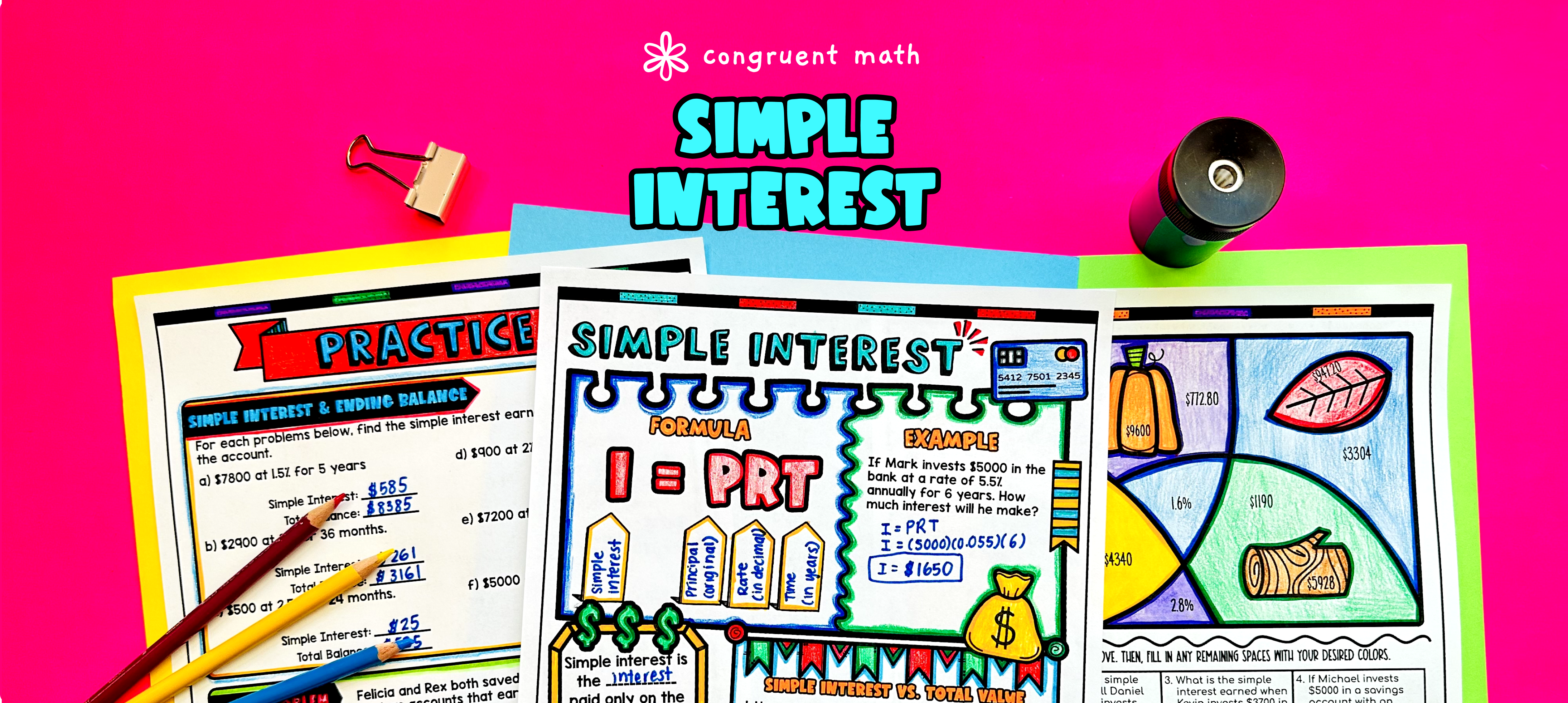Pin This

## Overview

Ever wondered how to teach simple interest in an engaging way to your seventh-grade students?

In this lesson plan, students will learn about calculating simple interest and its real-life applications. Through artistic and interactive guided notes, checks for understanding, a doodle and color by number activity, and a maze worksheet, students will gain a comprehensive understanding of simple interest.

The lesson culminates with a real-life example that explores the use of simple interest in car loans. Students will have the opportunity to read and write about how simple interest is used in this context, allowing them to see the practical application of the topic.

## Get the Lesson Materials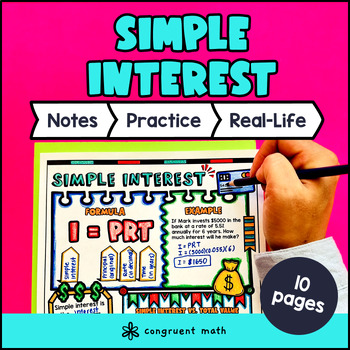\$4.25

## Learning Objectives

After this lesson, students will be able to:

• Define simple interest and identify its components (principal, rate, and time)

• Use the formula I = PRT to calculate simple interest, principal, or rate when given the other variables

• Solve multistep percent problems involving simple interest

• Apply the concept of simple interest to real-life situations, such as car loans.

## Prerequisites

Before this lesson, students should be familiar with:

• Basic multiplication and division of integers and decimals

• Knowledge of how to solve equations using substitution and inverse operations

## Key Vocabulary

• Simple interest

• Principal

• Total balance

• Percent

• Formula

• Rate

• Application

• Word problem

## Procedure

### Introduction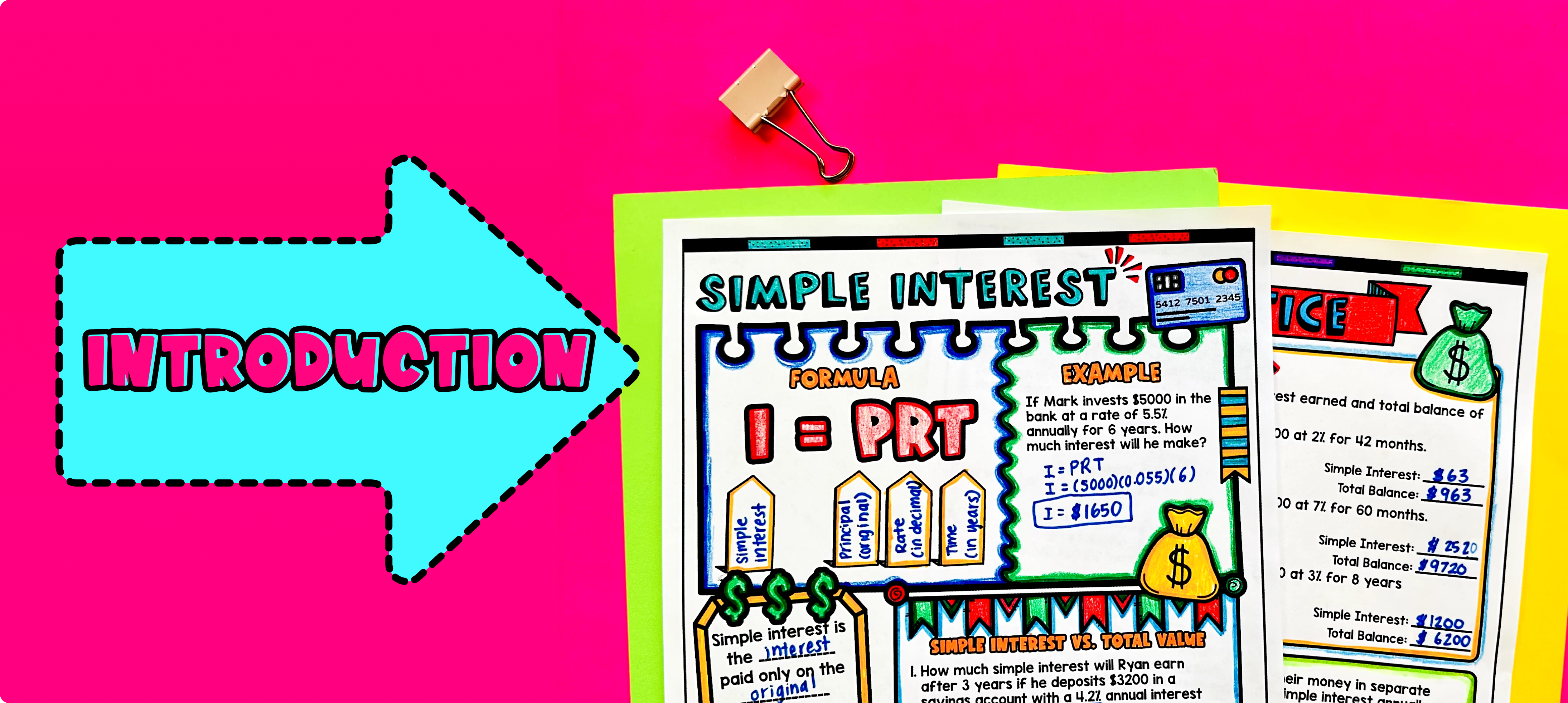Pin This

As a hook, ask students why understanding simple interest is important in real life financial situations. For example, you could ask them why it's important to know how much interest they will have to pay on a loan or how much they will earn in interest on a savings account. You can also refer to the last page of the guided notes and the FAQs for additional ideas.

Use the first page of the guided notes to introduce the topic of simple interest and the formula I = PRT. Explain that simple interest is the amount of money earned or paid on a principal amount over a certain period of time, and the formula allows us to calculate that amount. Walk through the key points of the formula and provide examples of how to use it to calculate simple interest. Use the first page of the guided notes to introduce the terms principal, rate, and time, and explain their role in the formula. Emphasize that the principal is the initial amount of money, the rate is the interest rate expressed as a decimal, and the time is the period over which the interest is calculated. Use the second page of the guided notes to explain the difference between calculating simple interest and total balance. Go through the word problems together.

Refer to the FAQ below for a walk-through on this, as well as ideas on how to respond to common student questions.

Based on student responses, reteach any concepts that students need extra help with. If your class has a wide range of proficiency levels, you can pull out students for reteaching, and have more advanced students begin working on the practice exercises.

### Practice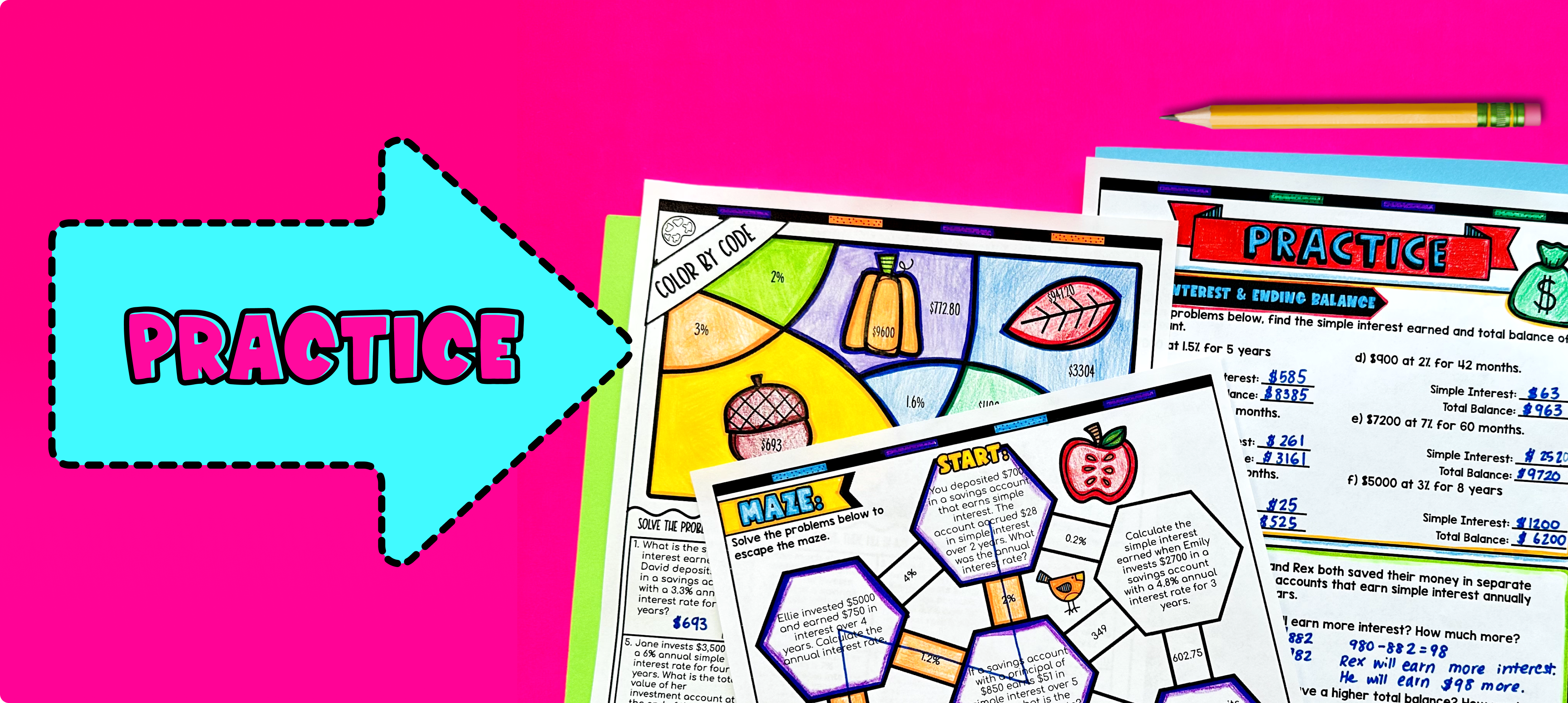Pin This

Have students practice calculating simple interest and total balance using the practice sheets in the guided notes resource (page 2). Walk around to answer student questions.

Fast finishers can dive into the maze activity (page 3) and color by number activity (page 4) for extra practice. You can assign it as homework for the remainder of the class.

### Real-Life Application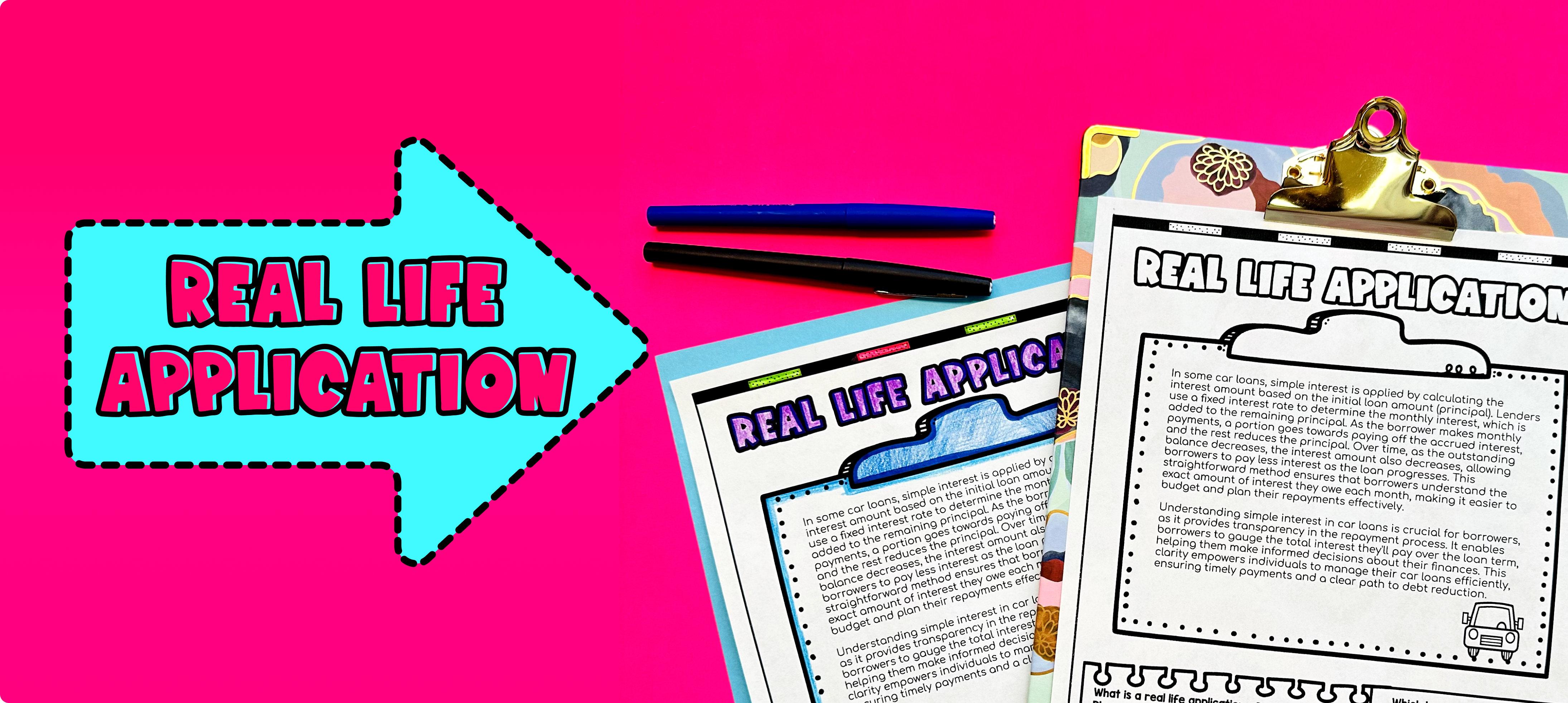Pin This

Bring the class back together, and introduce the concept of simple interest in real-life scenarios. Refer to the last page of the guided notes resource where students read about how simple interest is used in car loans.

Explain that simple interest is often used in financial situations such as loans, investments, and credit cards. It helps determine how much money is earned or paid for borrowing or investing a certain amount of money.

To provide a specific example, discuss the concept of car loans. Explain that when someone wants to buy a car but doesn't have enough money to pay for it upfront, they can take out a car loan from a bank or other financial institution. The bank will lend them the money to buy the car, but the borrower will have to pay back the loan over a period of time with additional interest.

Encourage students to share any personal experiences or brainstorm ideas related to loans and simple interest. This will help make the concept more relatable and engaging for them.

## Extensions

If you’re looking for digital practice for simple interest, try my Pixel Art activities in Google Sheets. Every answer is automatically checked, and correct answers unlock parts of a mystery picture. It’s incredibly fun, and a powerful tool for differentiation.

Here are 3 activities to explore: